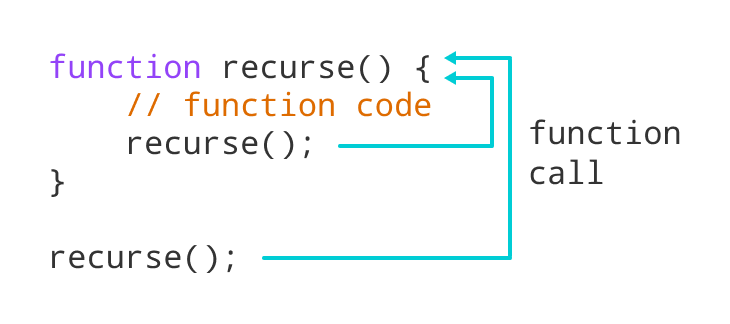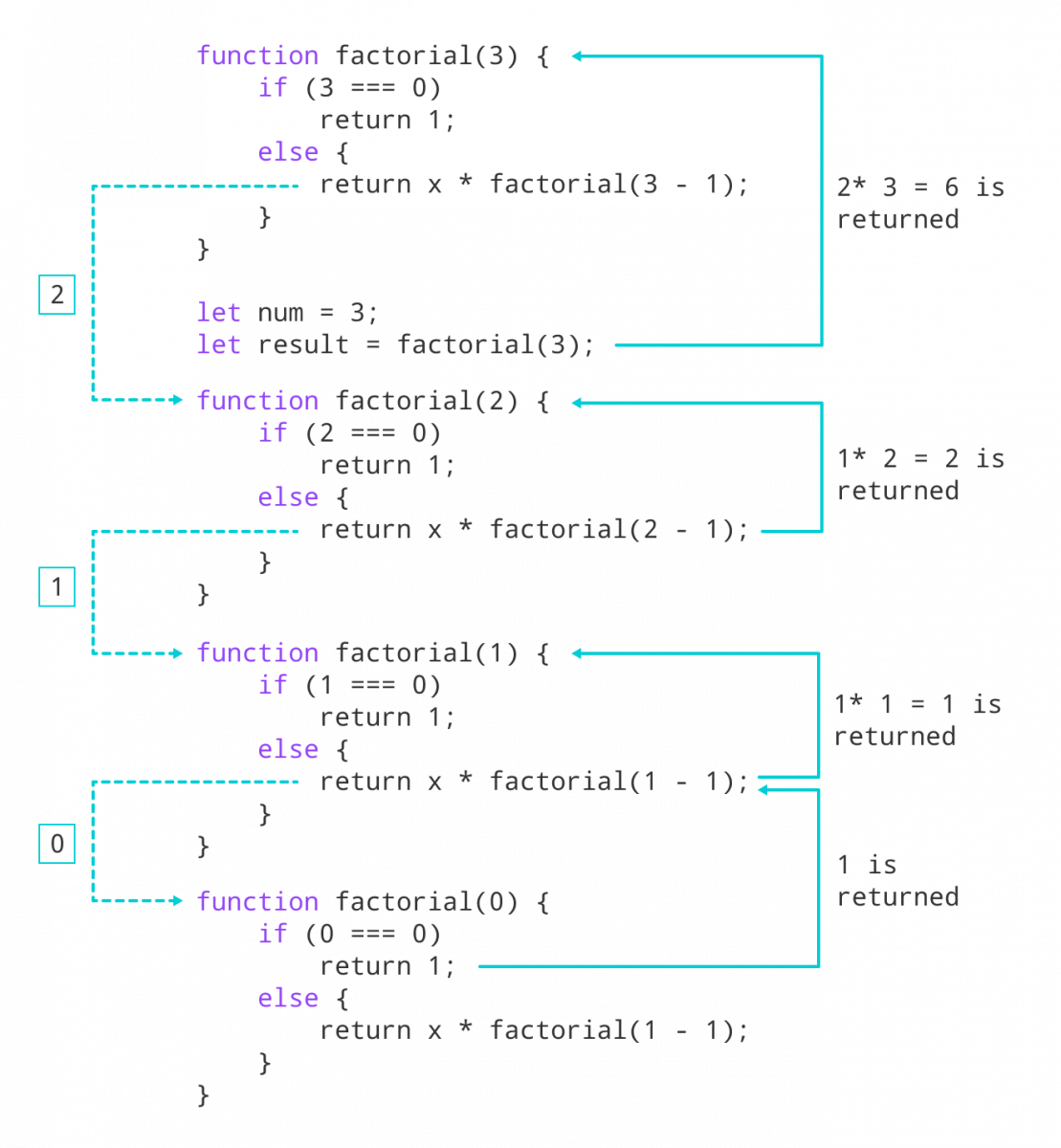# JavaScript Recursion

In this tutorial, you will learn about recursion in JavaScript with the help of examples.

Recursion is a process of calling itself. A function that calls itself is called a recursive function.

The syntax for recursive function is:

``````function recurse() {
// function code
recurse();
// function code
}

recurse();``````

Here, the `recurse()` function is a recursive function. It is calling itself inside the function.Working of recursion in JavaScript

A recursive function must have a condition to stop calling itself. Otherwise, the function is called indefinitely.

Once the condition is met, the function stops calling itself. This is called a base condition.

To prevent infinite recursion, you can use if...else statement (or similar approach) where one branch makes the recursive call, and the other doesn't.

So, it generally looks like this.

``````function recurse() {
if(condition) {
recurse();
}
else {
// stop calling recurse()
}
}

recurse();``````

A simple example of a recursive function would be to count down the value to 1.

``````// program to count down numbers to 1
function countDown(number) {

// display the number
console.log(number);

// decrease the number value
const newNumber = number - 1;

// base case
if (newNumber > 0) {
countDown(newNumber);
}
}

countDown(4);``````

Output

```4
3
2
1```

In the above program, the user passes a number as an argument when calling a function.

In each iteration, the number value is decreased by 1 and function `countDown()` is called until the number is positive. Here, `newNumber > 0` is the base condition.

This recursive call can be explained in the following steps:

```countDown(4) prints 4 and calls countDown(3)
countDown(3) prints 3 and calls countDown(2)
countDown(2) prints 2 and calls countDown(1)
countDown(1) prints 1 and calls countDown(0)```

When the number reaches 0, the base condition is met, and the function is not called anymore.

## Example 2: Find Factorial

``````// program to find the factorial of a number
function factorial(x) {

// if number is 0
if (x === 0) {
return 1;
}

// if number is positive
else {
return x * factorial(x - 1);
}
}

const num = 3;

// calling factorial() if num is non-negative
if (num > 0) {
let result = factorial(num);
console.log(`The factorial of \${num} is \${result}`);
}``````

Output

`The factorial of 3 is 6`

When you call function `factorial()` with a positive integer, it will recursively call itself by decreasing the number.

This process continues until the number becomes 1. Then when the number reaches 0, 1 is returned.Computing Factorial Using Recursion

This recursive call can be explained in the following steps:

```factorial(3) returns 3 * factorial(2)
factorial(2) returns 3 * 2 * factorial(1)
factorial(1) returns 3 * 2 * 1 * factorial(0)
factorial(0) returns 3 * 2 * 1 * 1```
Did you find this article helpful?